# Weak Aggregating Algorithm

Weak Aggregating Algorithm is one of the exponential weights algorithms, and has two differences with the Strong Aggregating Algorithm.
First is that it mixes losses without transfer them into an exponential space, so the formulae for generalized prediction in case of finite number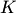of experts is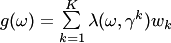, where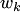are the weights of the experts,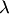is a loss function,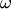are possible events. Second is that it uses a decreasing learning rate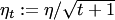to update the weights of the experts. It is clear, that one can use the same substitution function to provide a prediction. It has an advantage to provide theoretical bounds for weakly mixable functions (bounded functions), and even for unbounded loss functions. The theoretical bound for the loss of Weak Aggregating Algorithm is: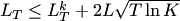for all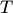and, where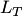is the loss suffered by master over the firsttrials, and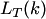is the loss suffered by the-th expert over the firsttrials. The best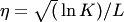. The constant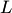is an upper bound of a loss function: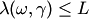.

### Bibliography

• Y.Kalnishkan and M.V.Vyugin. The weak aggregating algorithm and weak mixability. Journal of Computer and System Sciences, 74(8): 1228--1244 (2008).